# Pair of Straight Lines Solutions for exercise 4(a)

Intermediate first year mathematics 1b Pair of Straight Lines exercise 4(a) solutions are given.

Study the textbook lesson Pair Straight Lines very well.

Observe the example problems and solutions given in the textbook.

Read the Key points number of times.

Observe the solutions and try them in your own method.

You can also see

SSC Maths text book Solutions class 10

Inter Maths 1A Solutions textbook

Inter Maths IIB text book solutions

The Straight Line

Exercise 3(a)

Exercise 3(b)

Exercise 3(c)

Exercise 3(d)

Exercise 3(e)

M

# Exercise 4(a) solutions inter maths 1b

Chapter – 4 Pair of Straight Lines

Exercise 4(a)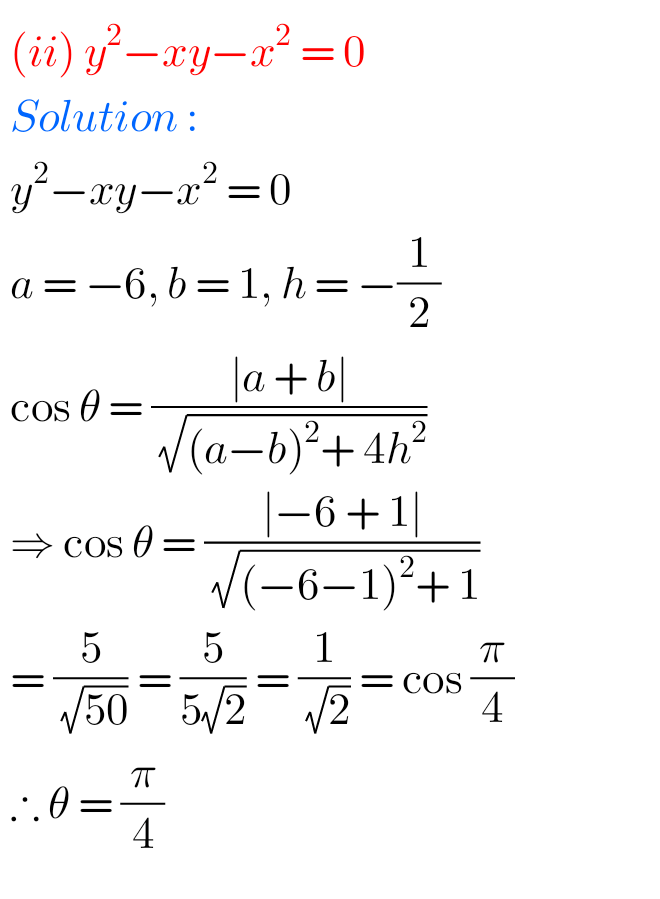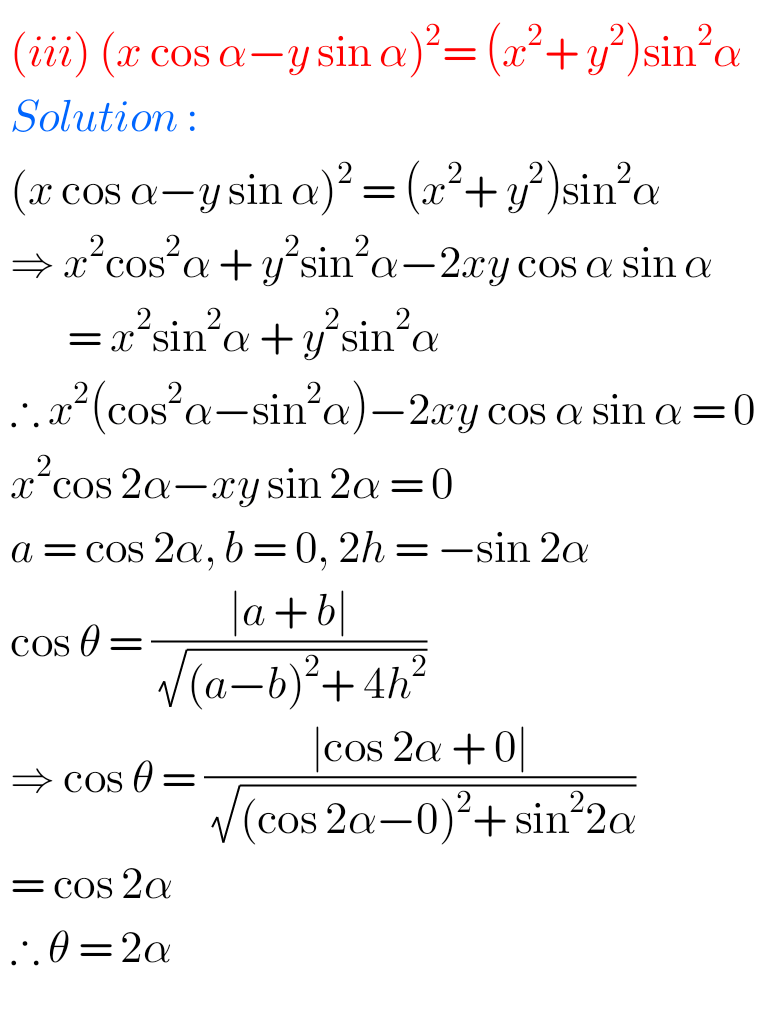iv.

M

## Solutions for Pair of Straight Lines exercise 4(a)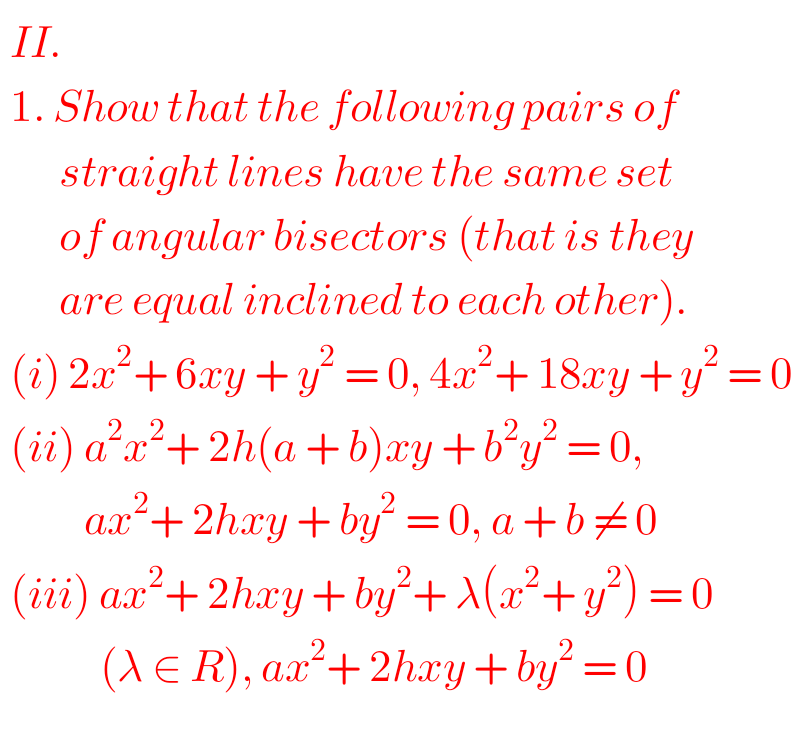F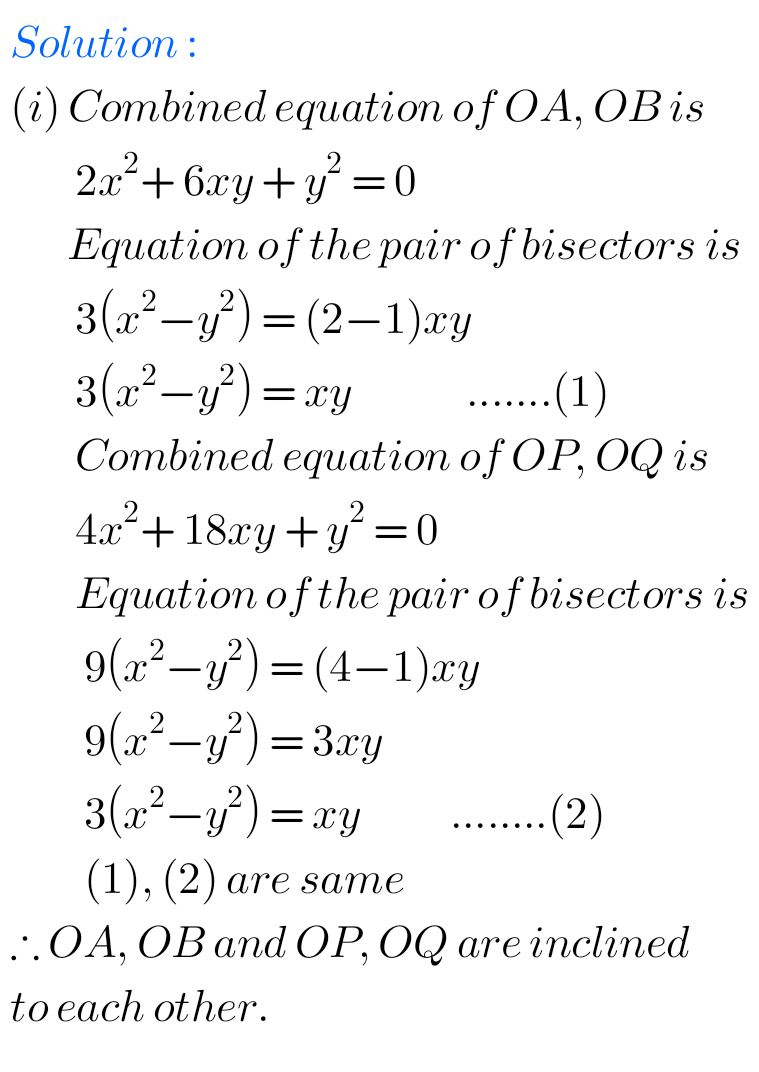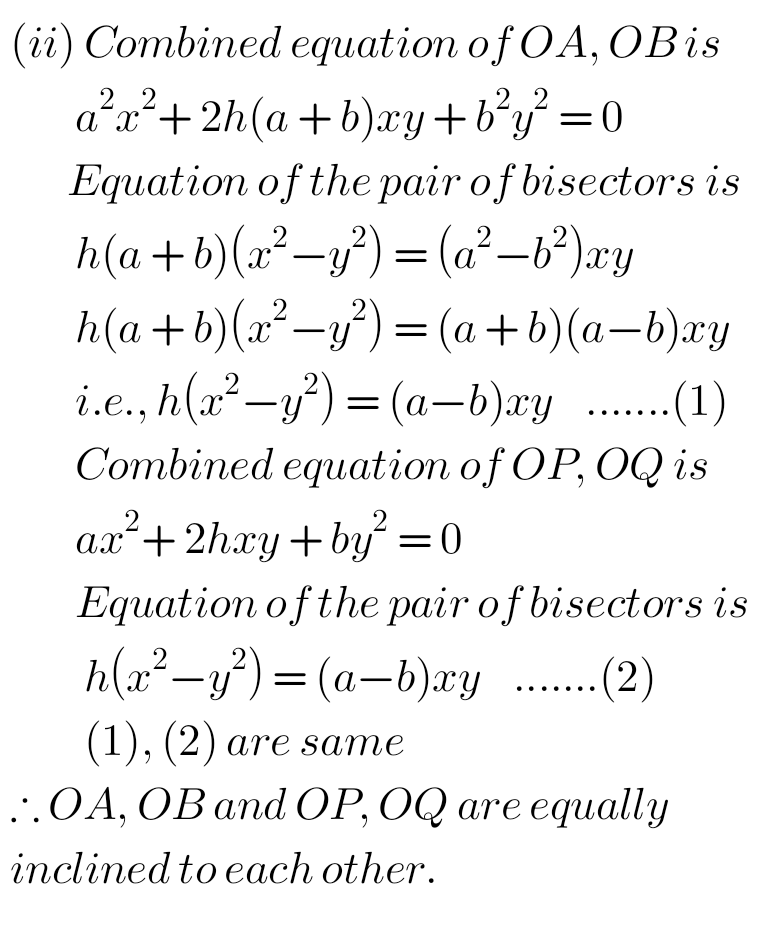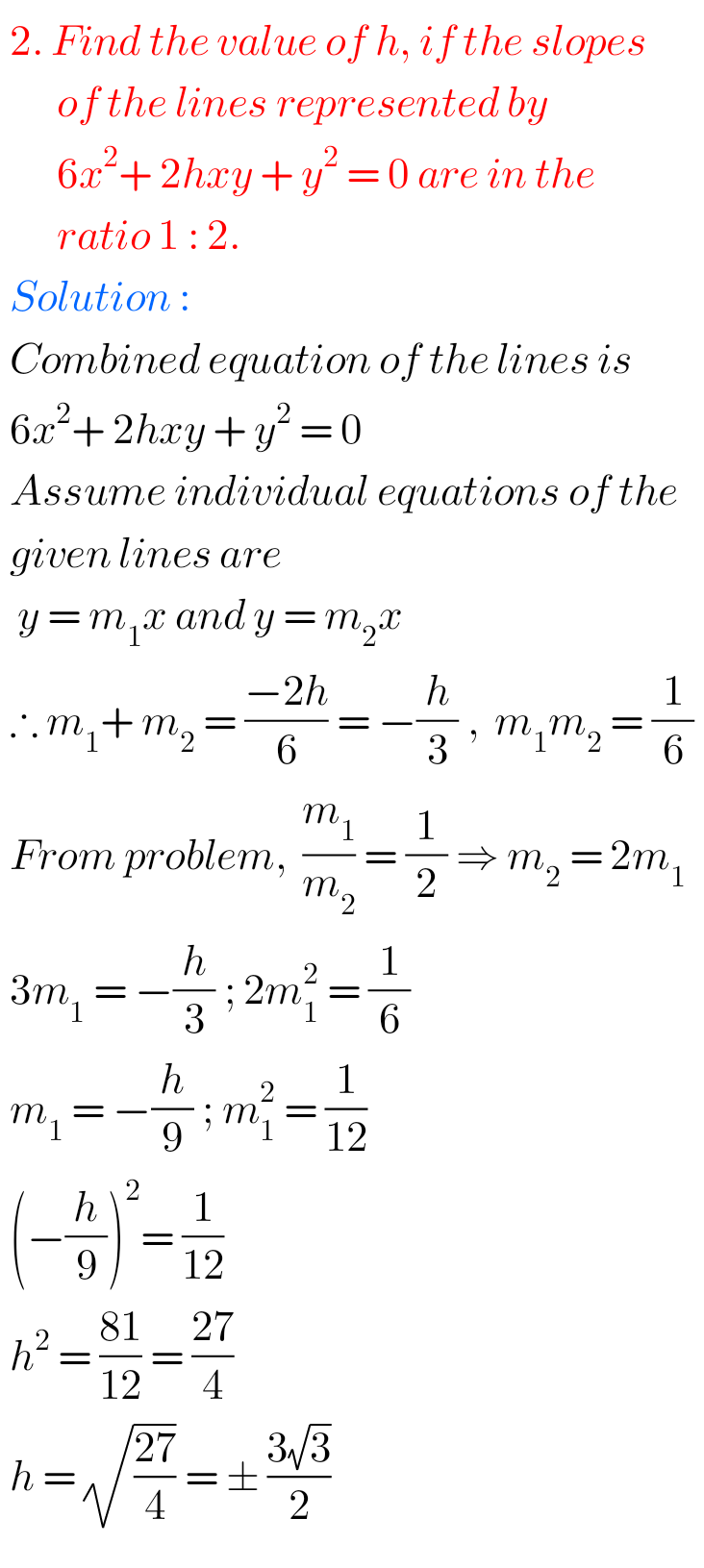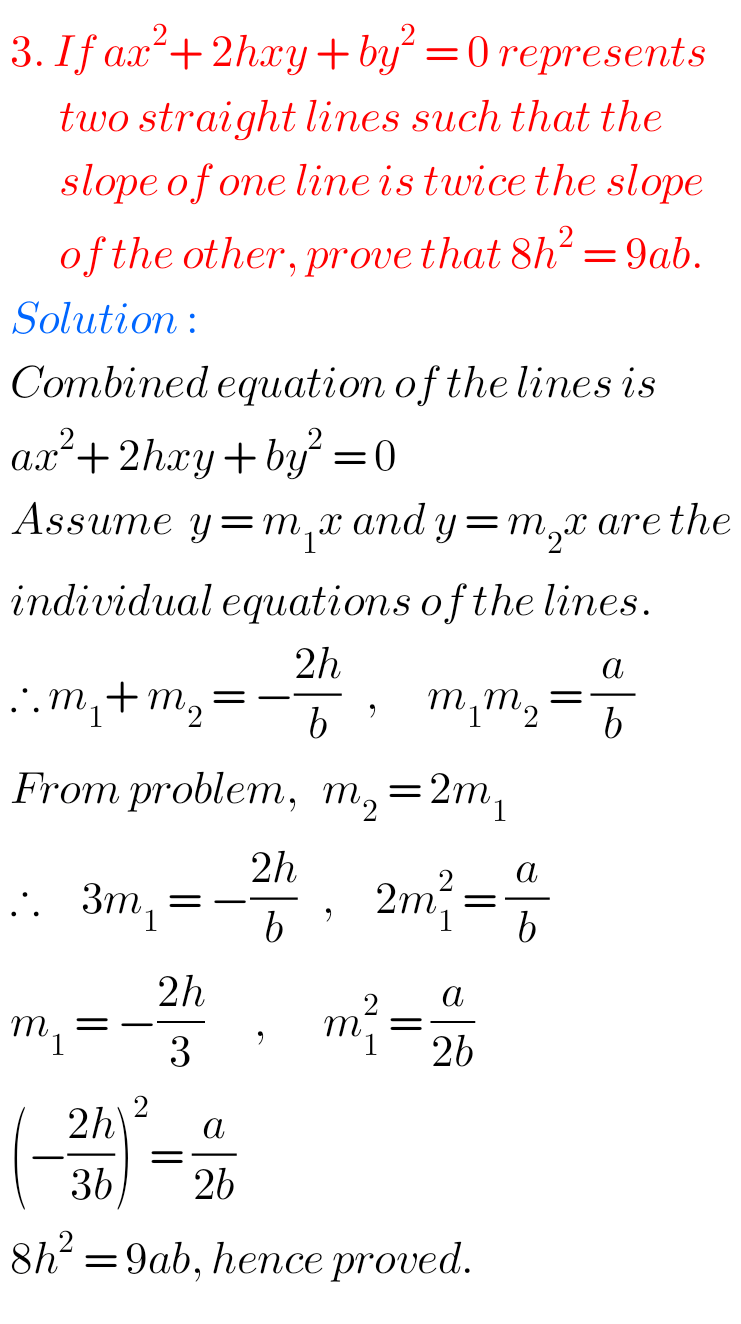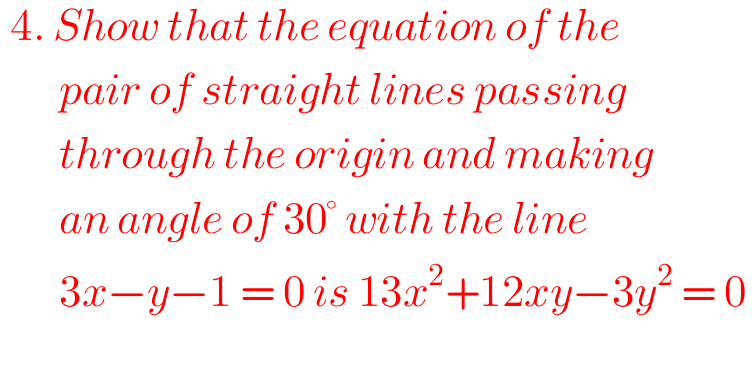F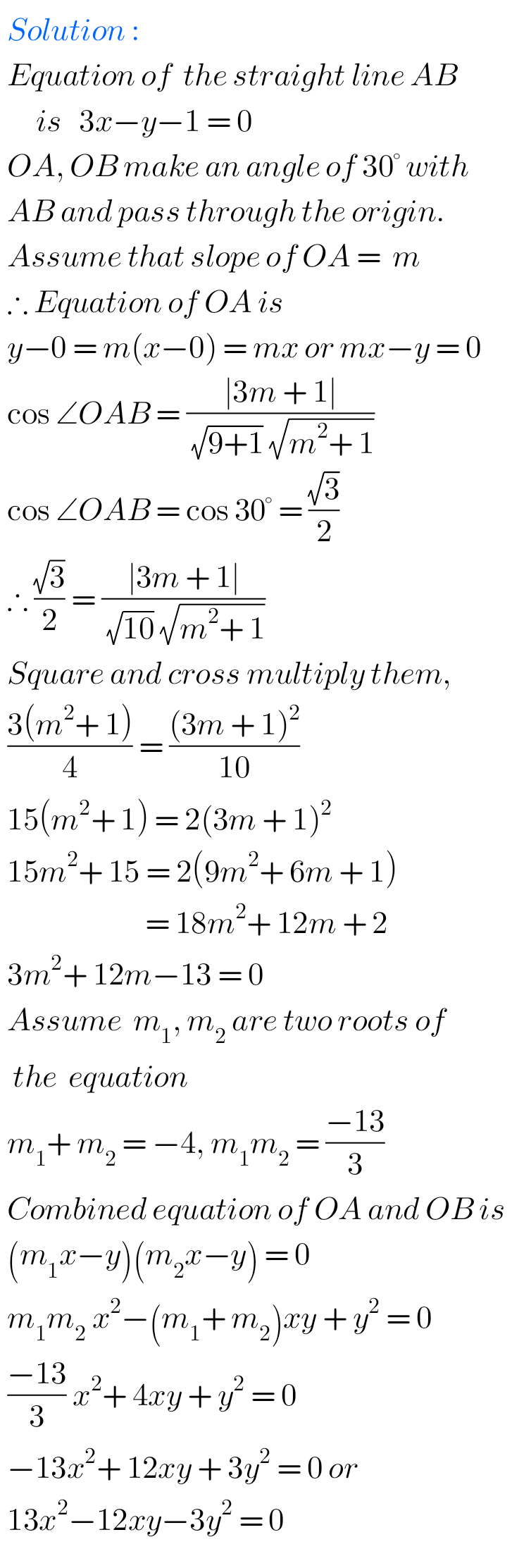F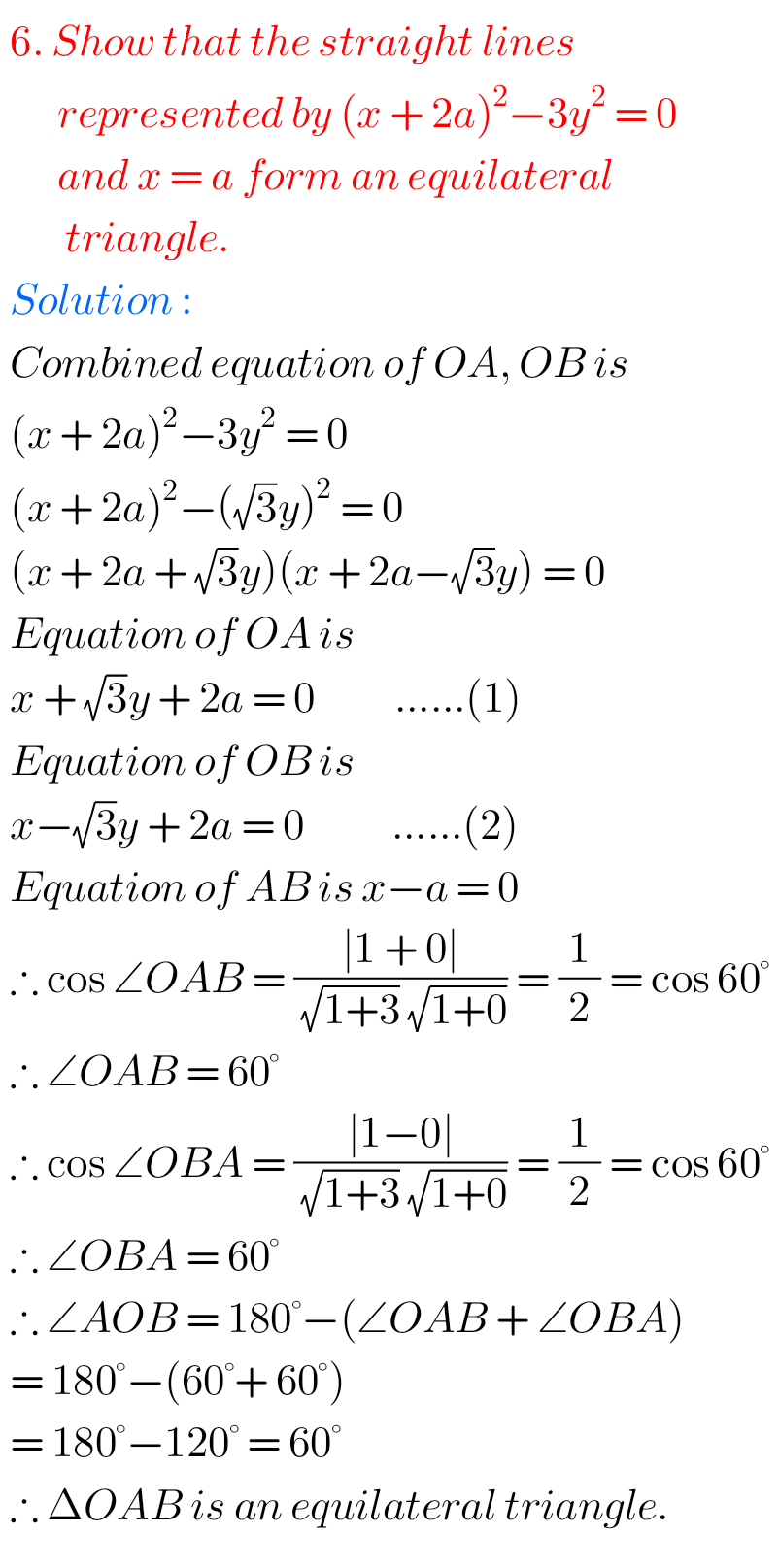F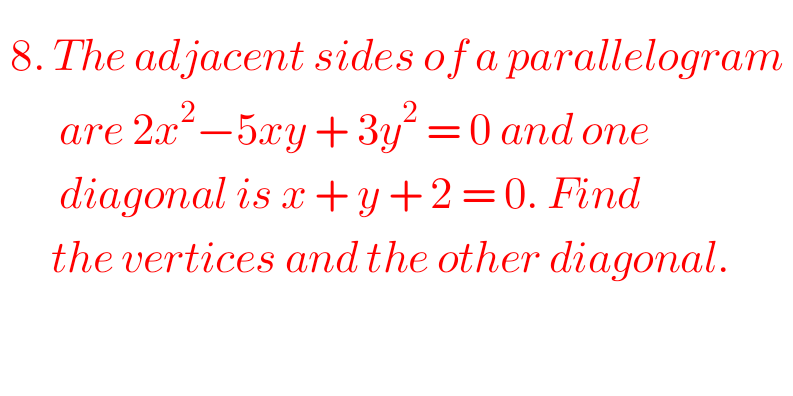F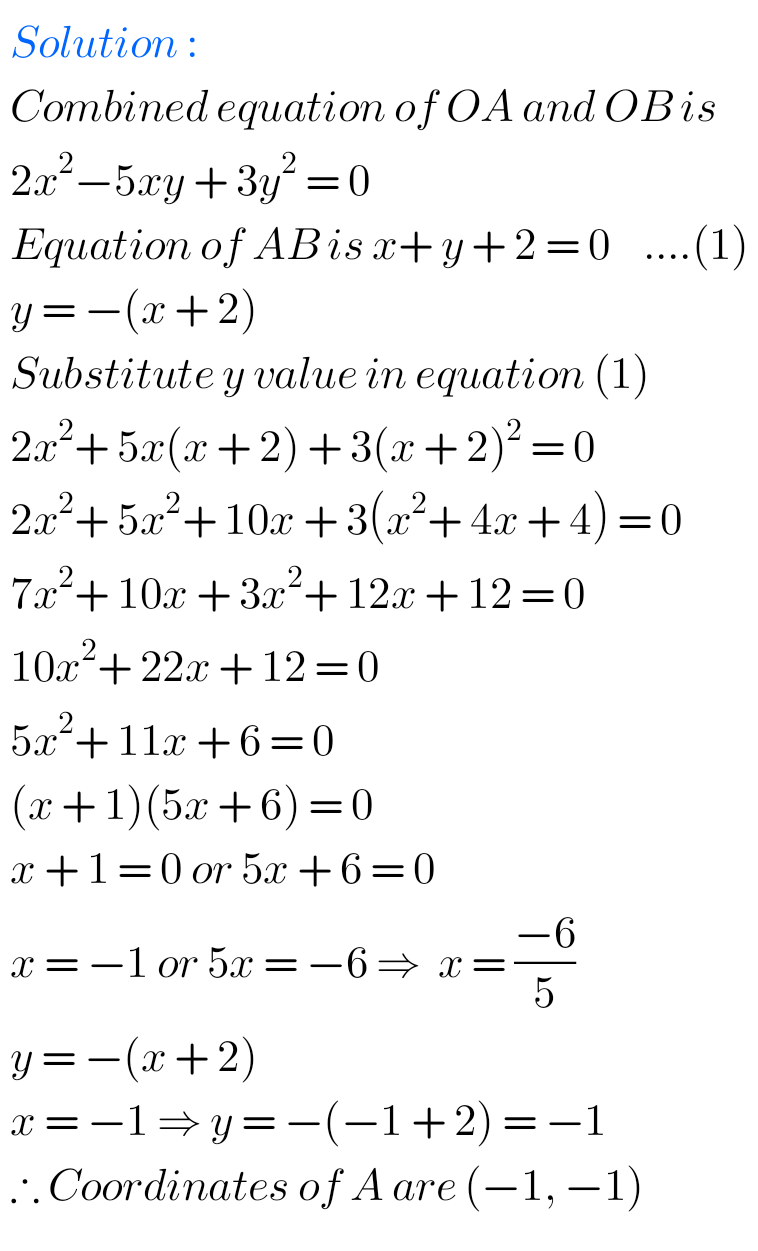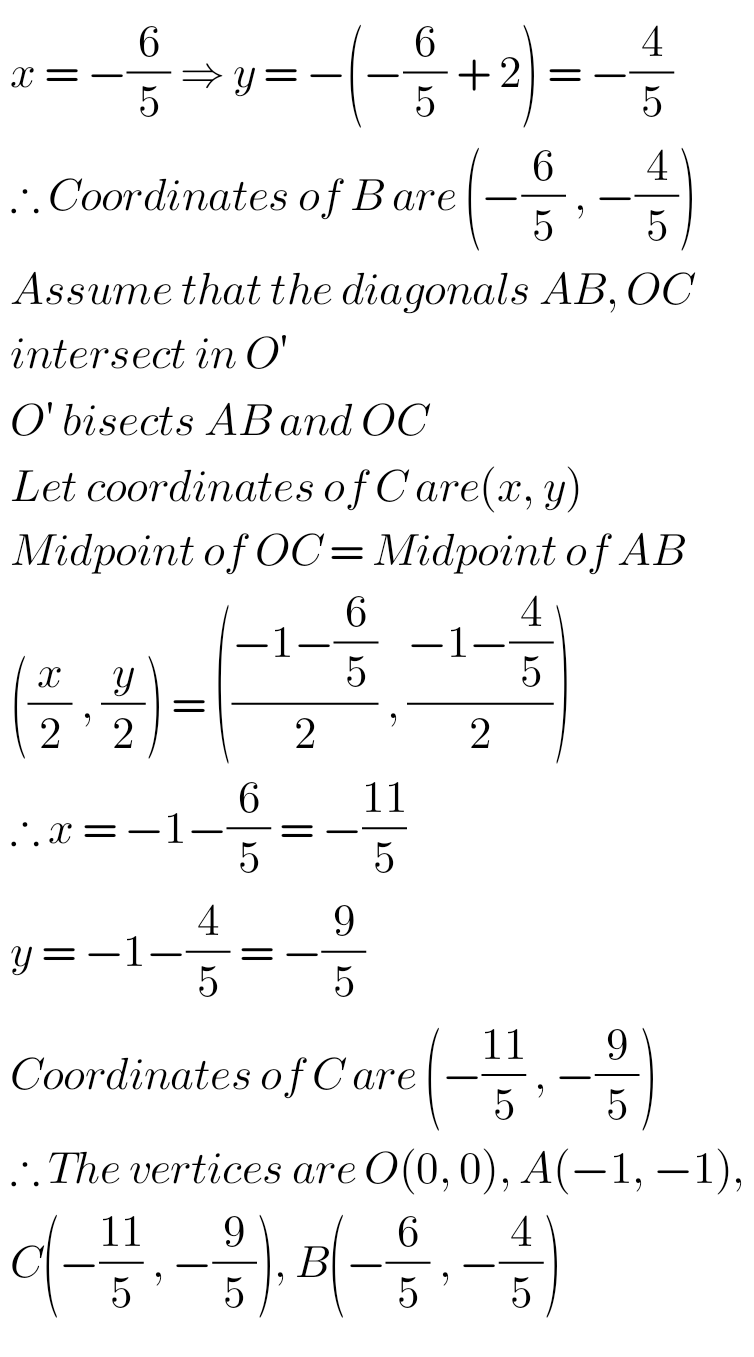FFF11.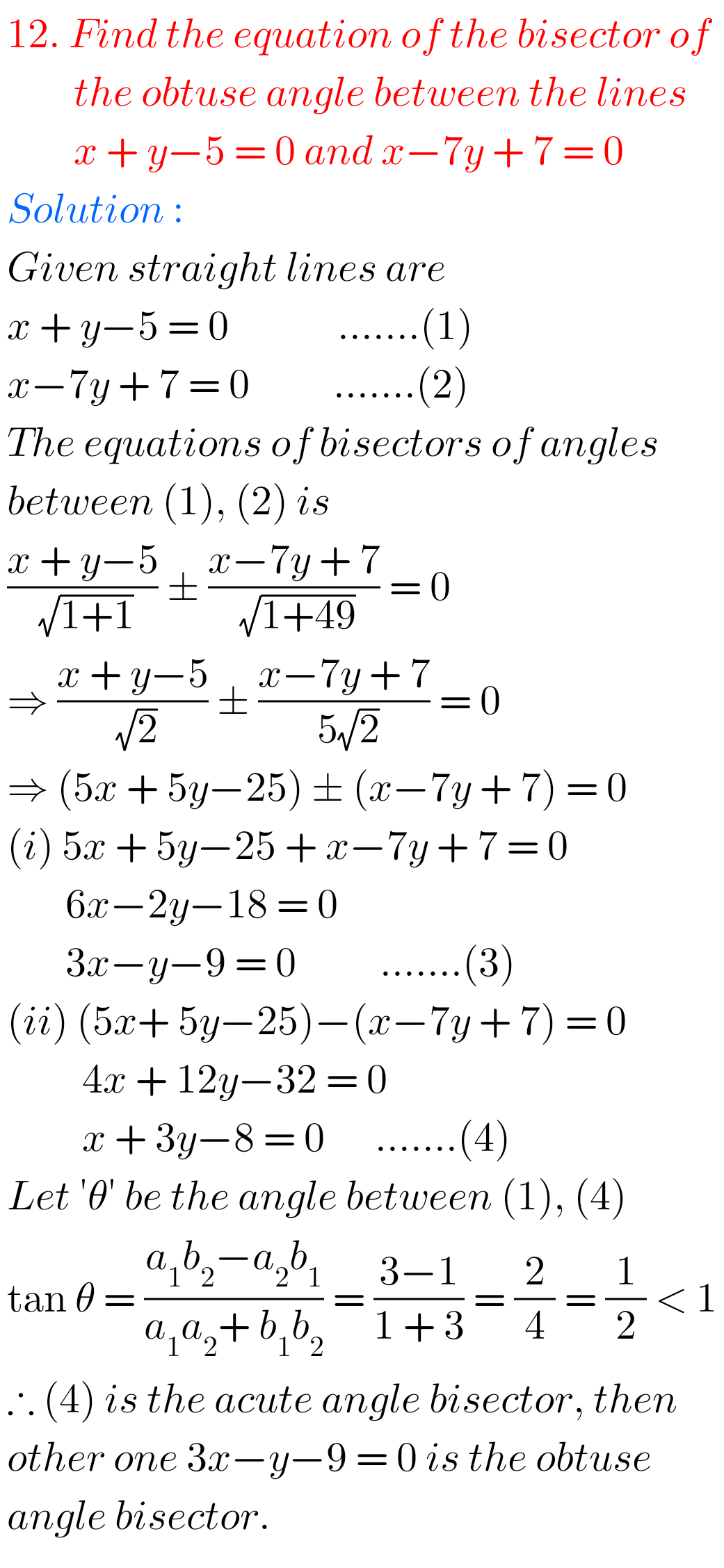M

## Intermediate 1st year Maths 1b Pair of Straight Lines Exercise 4(a) solutions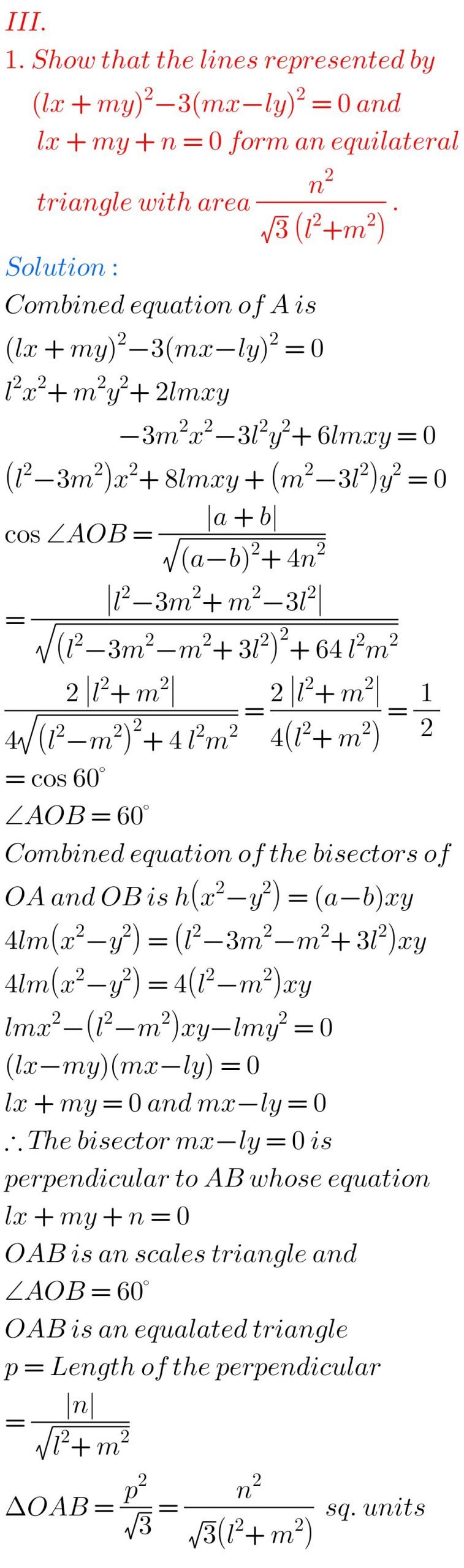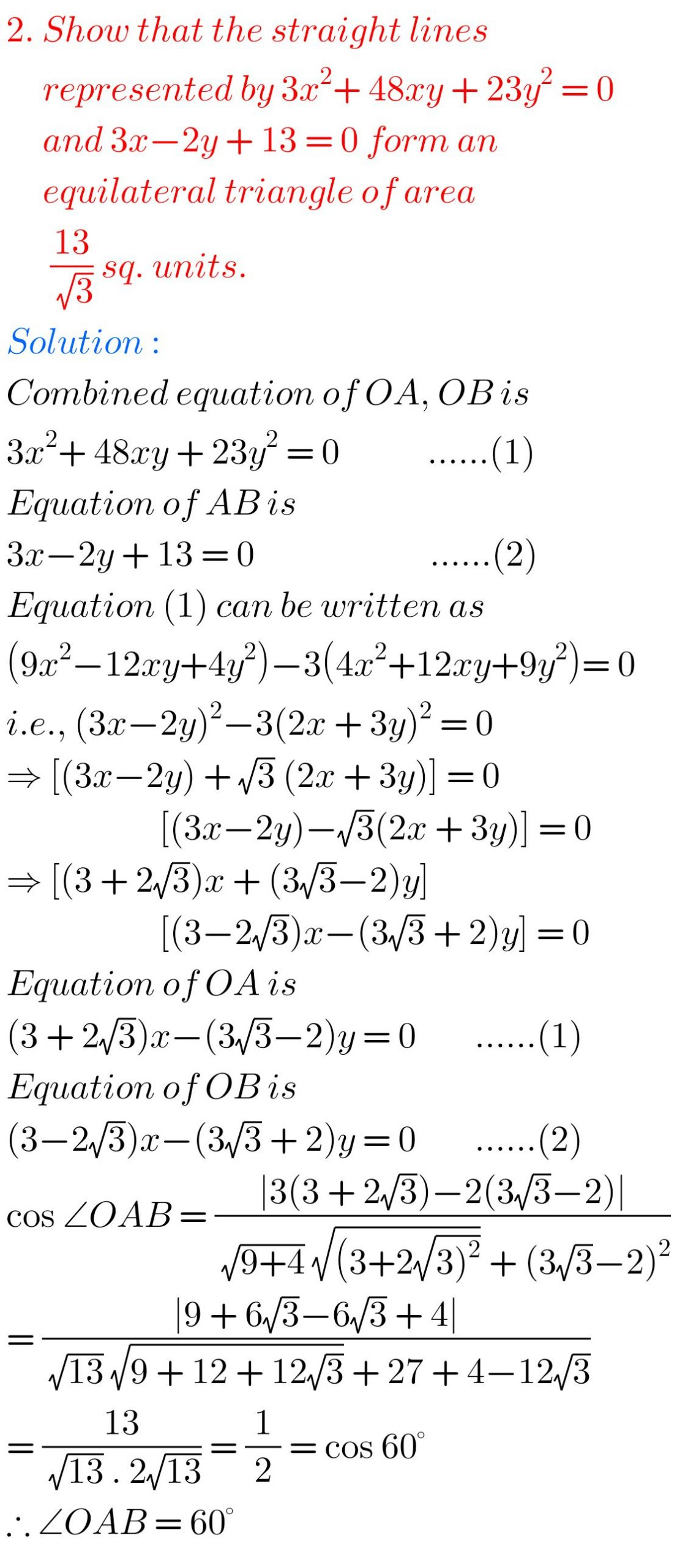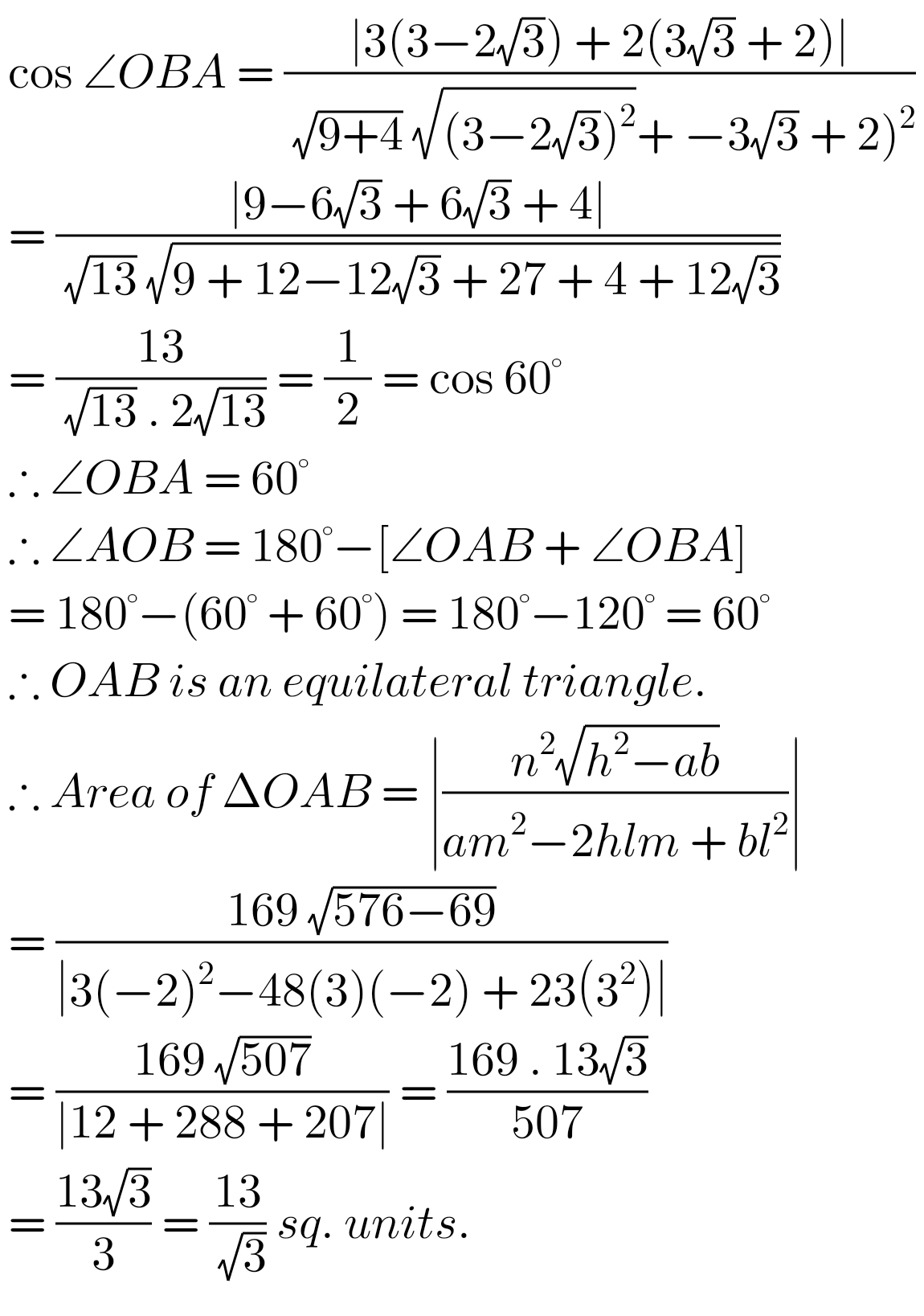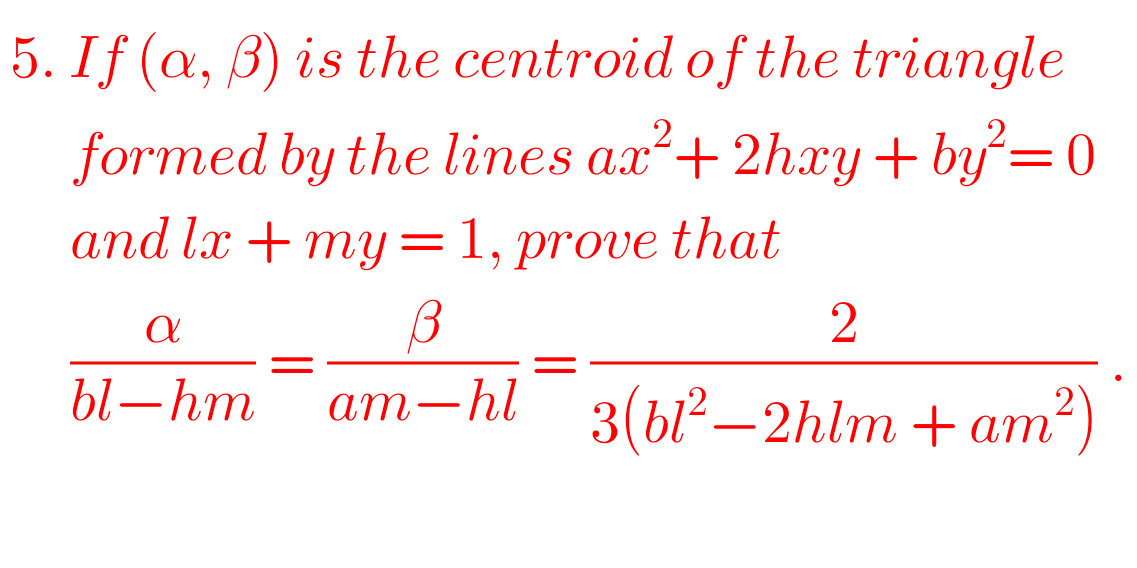FF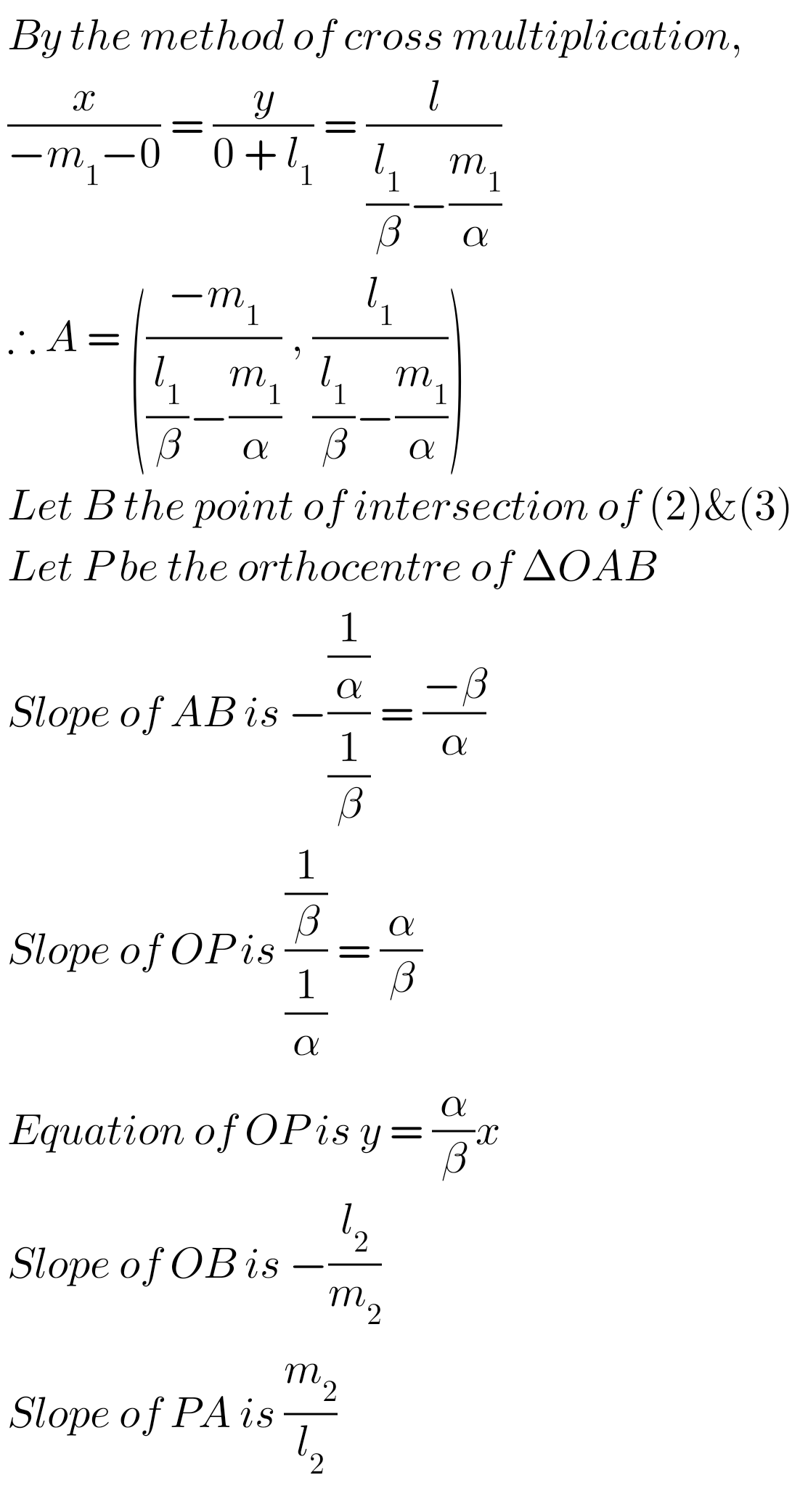F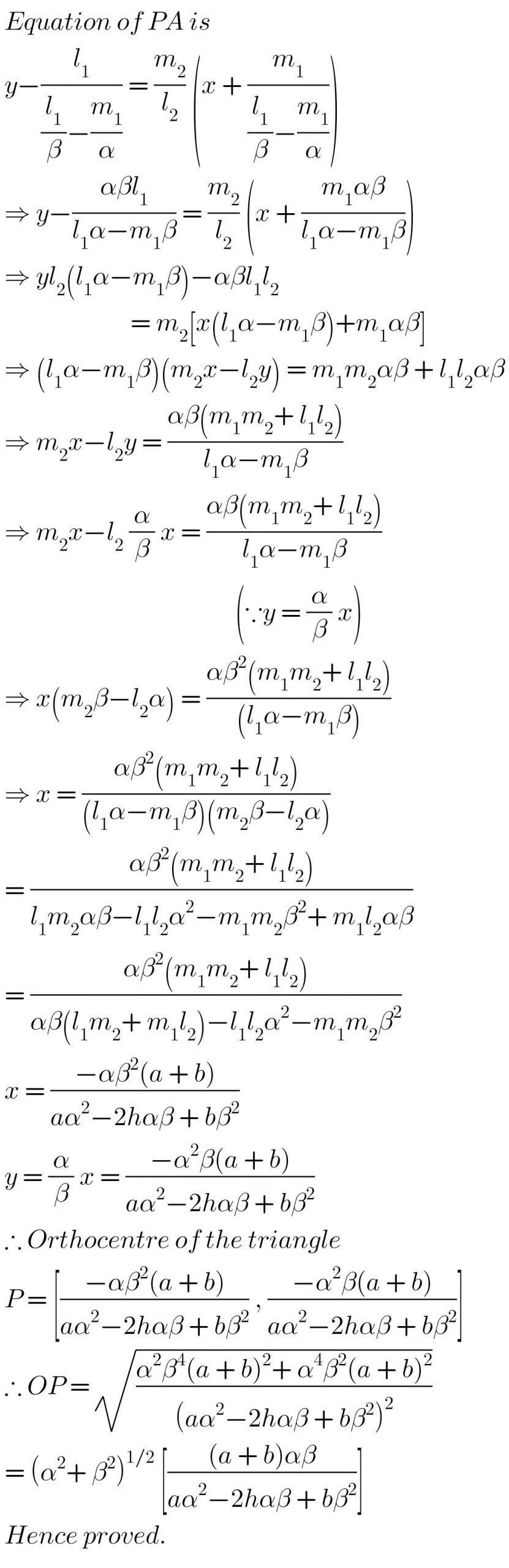FNote : Observe the solutions and try them in your own method.

Ncert maths class 6 chapter 14 solutions

Nios maths 311 book 1 Sets 1.1 solutions